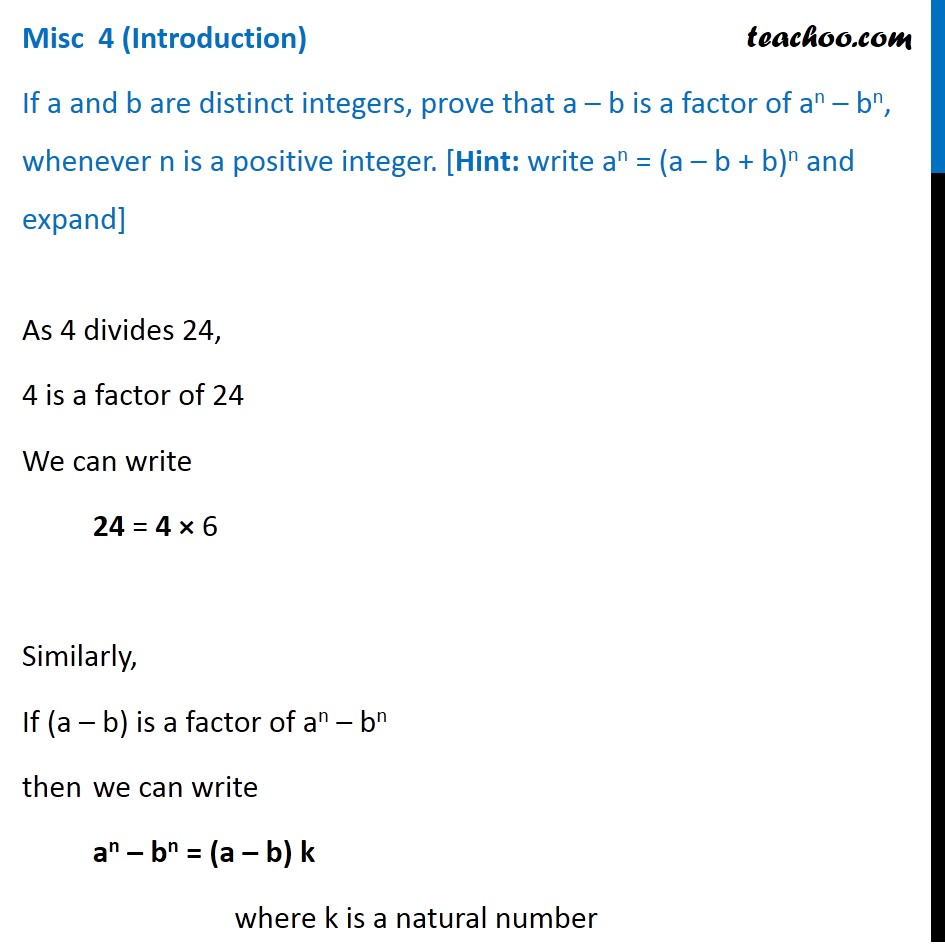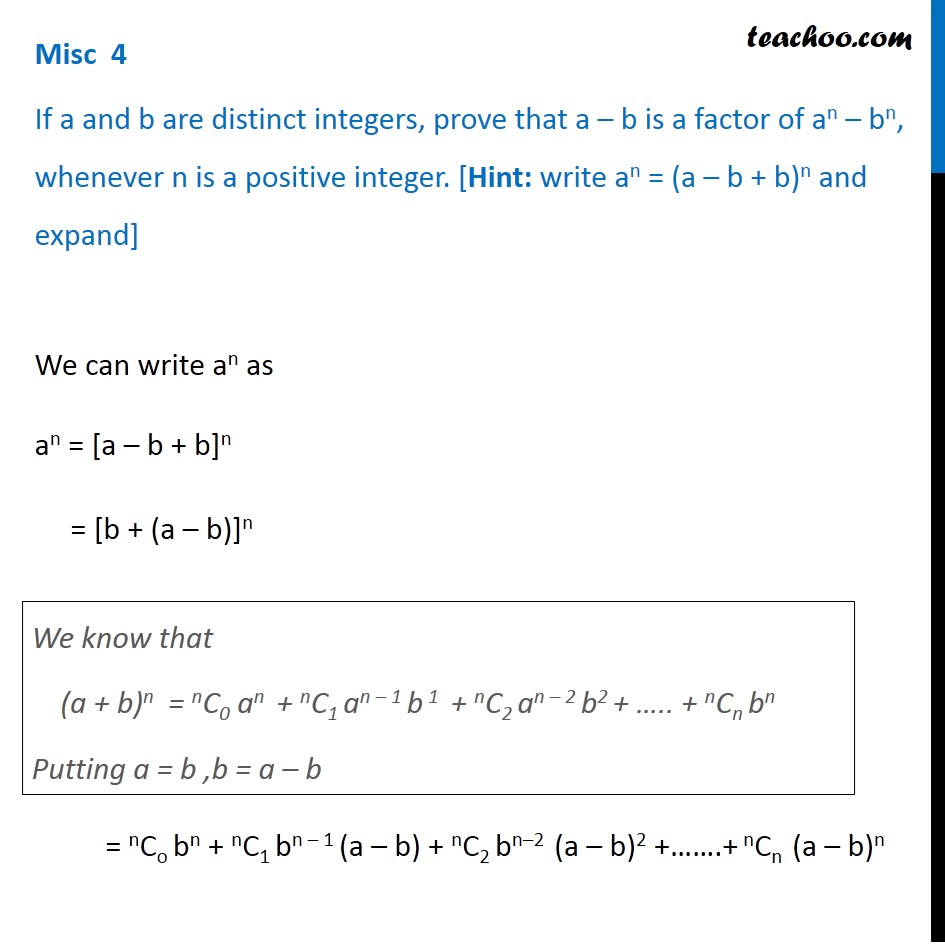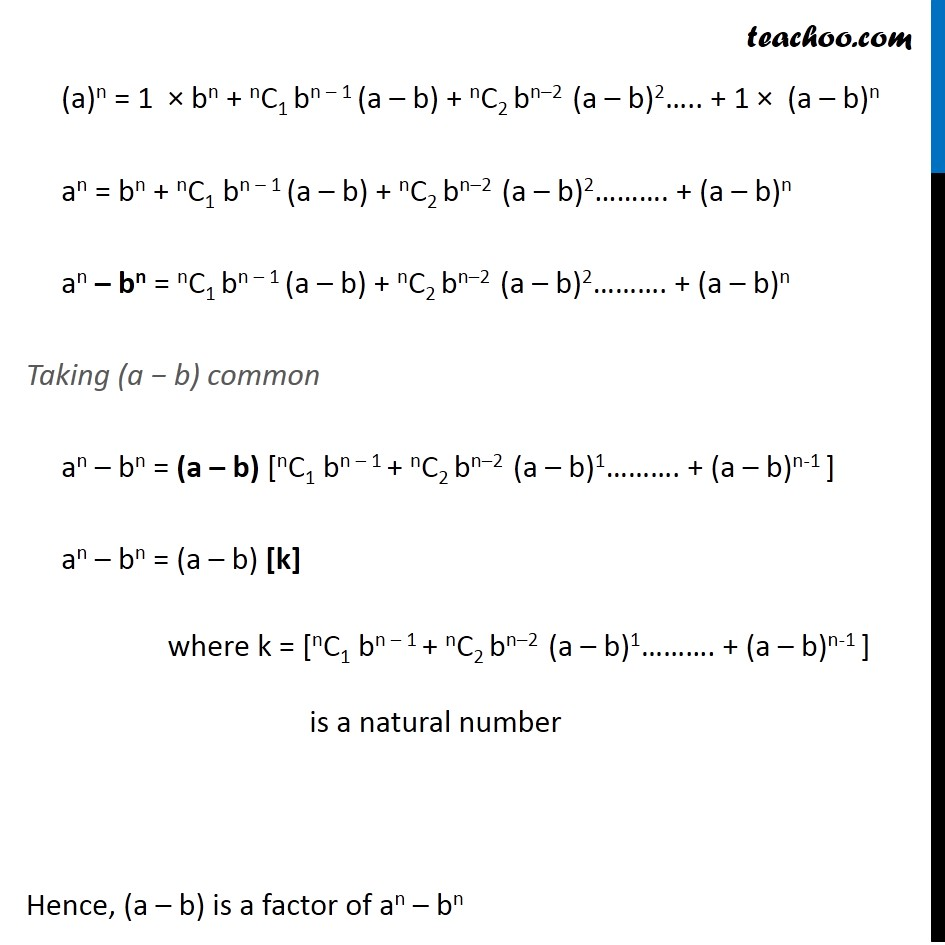Miscellaneous

Chapter 7 Class 11 Binomial Theorem
Serial order wiseLearn in your speed, with individual attention - Teachoo Maths 1-on-1 Class

### Transcript

Misc 1 (Introduction) If a and b are distinct integers, prove that a – b is a factor of an – bn, whenever n is a positive integer. [Hint: write an = (a – b + b)n and expand] As 4 divides 24, 4 is a factor of 24 We can write 24 = 4 × 6 Similarly, If (a – b) is a factor of an – bn then we can write an – bn = (a – b) k where k is a natural number Misc 1 If a and b are distinct integers, prove that a – b is a factor of an – bn, whenever n is a positive integer. [Hint: write an = (a – b + b)n and expand] We can write an as an = [a – b + b]n = [b + (a – b)]n = nCo bn + nC1 bn – 1 (a – b) + nC2 bn–2 (a – b)2 +…….+ nCn (a – b)n We know that (a + b)n = nC0 an + nC1 an – 1 b 1 + nC2 an – 2 b2 + ….. + nCn bn Putting a = b ,b = a – b (a)n = 1 × bn + nC1 bn – 1 (a – b) + nC2 bn–2 (a – b)2….. + 1 × (a – b)n an = bn + nC1 bn – 1 (a – b) + nC2 bn–2 (a – b)2………. + (a – b)n an – bn = nC1 bn – 1 (a – b) + nC2 bn–2 (a – b)2………. + (a – b)n Taking (a − b) common an – bn = (a – b) [nC1 bn – 1 + nC2 bn–2 (a – b)1………. + (a – b)n-1 ] an – bn = (a – b) [k] where k = [nC1 bn – 1 + nC2 bn–2 (a – b)1………. + (a – b)n-1 ] is a natural number Hence, (a – b) is a factor of an – bn